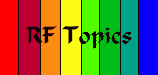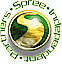Spread Spectrum SceneThe Decibel Defined The Decibel is the unit used to express relative differences in signal strength. It is expressed as the base 10 logarithm of the ratio of the powers of two signals: dB = 10 log (P1/P2) Signal amplitude can also be expressed in dB. Since power is proportional to the square of a signal's amplitude (e.g., a power ratio of 100 is equivalent to an amplitude ratio of 10), dB is expressed as follows: dB = 20 log (A1/A2) Logarithms are useful as the unit of measurement because (1) signal power tends to span several orders of magnitude and (2) signal attenuation losses and gains can be expressed in terms of subtraction and addition. For example, suppose that a signal that passes through two channel segments is first attenuated in the ratio of 20 to 1 on the first leg and 7 to 1 on the second. The total signal degradation is in the ratio of 140 to 1. Expressed in dB, this becomes 13.01 (10 log 20) + 8.45 (10 log 7) = 21.46 dB. The following table helps to indicate the order of magnitude associated with dB: 1 dB attenuation means that 0.79 of the input power survives 3 dB attenuation means that 0.50 of the input power survives 10 dB attenuation means that 0.1 of the input power survives 20 dB attenuation means that 0.01 of the input power survives 30 dB attenuation means that 0.001 of the input power survives 40 dB attenuation means that 0.0001 of the input power survives NOTE: Many times dB ratios are expressed using a third letter (or letters), e.g. dBm -- the third letter is a reference level for the log operation. For instance, dBm is used to define dB levels in a 50 Ohm RF system, using a 1 milliwatt reference level. Thanks: Randy H. Katz, randy@cs.Berkeley.eduShop at Spree.com!